## RD Sharma Class 9 Solutions Chapter 1 Number Systems MCQS

These Solutions are part of RD Sharma Class 9 Solutions. Here we have given RD Sharma Class 9 Solutions Chapter 1 Number Systems MCQS

Other Exercises

the correct alternative in each of the following:
Question 1.
Which one of the following is a correct statement?
(a) Decimal expansion of a rational number is terminating
(b) Decimal expansion of a rational number is non-terminating
(c) Decimal expansion of an irrational number is terminating
(d) Decimal expansion of an irrational number is non-terminating and non-repeating
Solution:
Decimal expansion of an irrational number is non-terminating and non-repeating . (d)

Question 2.
Which one of the following statements is true?
(a) The sum of two irrational numbers is always an irrational-number
(b) The sum of two irrational numbers is always a rational number
(c) The sum of two irrational numbers may be a rational number or an irrational number
(d) The sum of two irrational numbers is always an integer
Solution:
The sum of two irrational numbers may be a rational number or an irrational number (c)

Question 3.
Which of the following is a correct statement?
(a) Sum of two irrational numbers is always irrational
(b) Sum of a rational and irrational number is always an irrational number
(c) Square of an irrational number is always a rational number
(d) Sum of two rational numbers can never be an integer
Solution:
Sum of a rational and irrational number is always an irrational number         (b)

Question 4.
Which of the following statements is true?
(a) Product of two irrational numbers is always irrational
(b) Product of a rational and an irrational number is always irrational
(c) Sum of two irrational numbers can never be irrational
(d) Sum of an integer and a rational number can never be an integer
Solution:
Product of a rational and an irrational number is always irrational    (b)

Question 5.
Which of the following is irrational?Solution: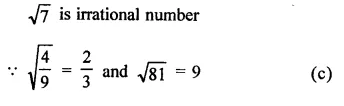Question 6.
Which of the following is irrational?
(a) 14
(b)  0.14$$\overline { 16 }$$
(c)   0.$$\overline { 1416 }$$
(d)  0.1014001400014
Solution:
0.1014001400014…….. is irrational as it is non-terminating nor repeating decimal, (d)

Question 7.
Which of the following is rational?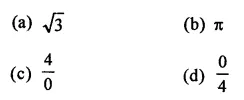Solution:Question 8.
The number 0.318564318564318564… is:
(a) a natural number
(b) an integer
(c) a rational number
(d) an irrational number
Solution:
The number = 0.318564318564318564…………
= 0.$$\overline { 318564 }$$
∵ The decimal is non-terminating and recurring
∴ It is rational number.   (c)

Question 9.
If n is a natural number, then $$\sqrt { n }$$is
(a) always a natural number
(b) always a rational number
(c) always an irrational number
(d) sometimes a natural number and sometimes an irrational number
Solution:
If n is a natural number then $$\sqrt { n }$$ may sometimes a natural number and sometime an irrational number e.g.
If n = 2 then $$\sqrt { n }$$ =$$\sqrt { 2 }$$ which is are irrational and if n = 4, then $$\sqrt { n }$$= $$\sqrt { 4 }$$ =  2 which is a rational number.       (d)

Question 10.
Which of the following numbers can be represented as non-terminating, repeating decimals?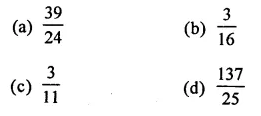Solution:Question 11.
Every point on a number line represents
(a) a unique real number
(b) a natural number
(c) a rational number
(d) an irrational number
Solution:
Every point on a number line represents a unique real number.         (a)

Question 12.
Which of the following is irrational?
(a) 0.15
(b) 0.01516
(c) 0.$$\overline { 1516 }$$
(d) 0.5015001500015..
Solution:
As it is non-terminating non-repeating decimals while others are terminating or non-terminating repeating decimals. (d)

Question 13.
The number 1.$$\overline { 27 }$$ in the form $$\frac { p }{ q }$$  , where p and q are integers and q ≠ 0, isSolution: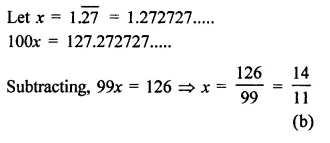Question 14.Solution: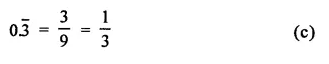Question 15.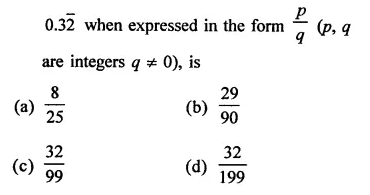Solution:Question 16.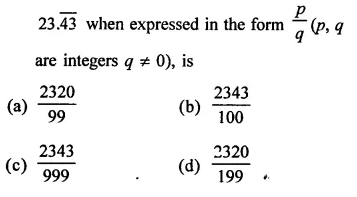Solution:Question 17.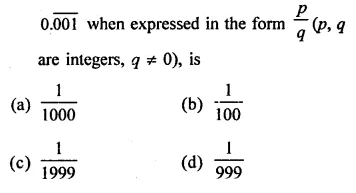Solution: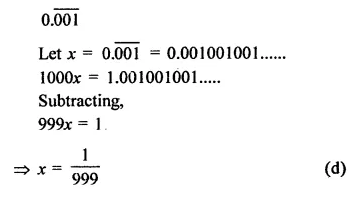Question 18.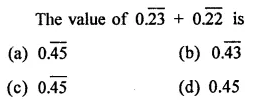Solution: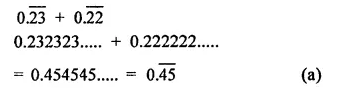Question 19.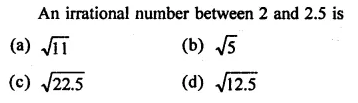Solution:
An irrational number between 2 and 2.5 is $$\sqrt { 5 }$$ as it has approximate value 2.236… (b)

Question 20.
The number of consecutive zeros in 23 x 34 x 54 x 7, is
(a) 3
(b) 2
(c) 4
(d) 5
Solution:
In 23 x 34 x 54 x 7, number of consecutive zero will be 3 as 23 x 54 = 2 x 2 x 2 x 5x 5 x 5 x 5 = 5000      (a)

Question 21.
The smallest rational number by which $$\frac { 1 }{ 3 }$$ should be multiplied so that its decimal expansion terminates after one place of decimal, is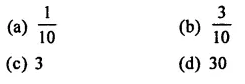Solution:Hope given RD Sharma Class 9 Solutions Chapter 1 Number Systems MCQS are helpful to complete your math homework.

If you have any doubts, please comment below. Learn Insta try to provide online math tutoring for you.